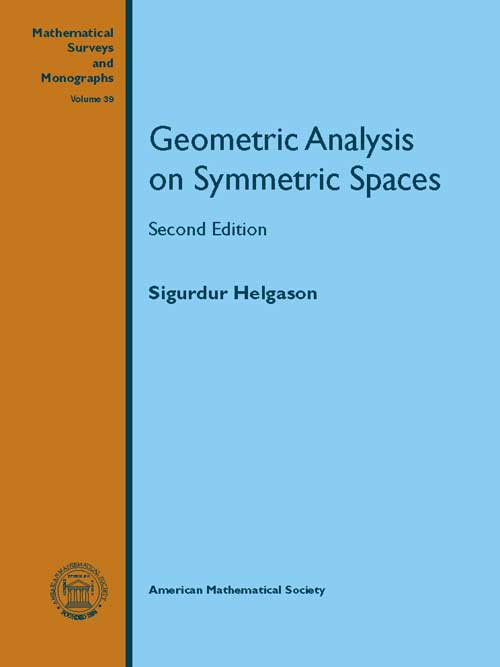Geometric Analysis on Symmetric Spaces
Sigurdur Helgason
Price
2025.00
ISBN
9780821868959
Language
English
Pages
656
Format
Paperback
Dimensions
180 x 240 mm
Year of Publishing
2011
Territorial Rights
Restricted
Imprint
American Mathematical Society
Catalogues

This book gives the first systematic exposition of geometric analysis on Riemannian symmetric spaces and its relationship to the representation theory of Lie groups. The book starts with modern integral geometry for double fibrations and treats several examples in detail. After discussing the theory of Radon transforms and Fourier transforms on symmetric spaces, inversion formulas, and range theorems, Helgason examines applications to invariant differential equations on symmetric spaces, existence theorems, and explicit solution formulas, particularly potential theory and wave equations. The canonical multitemporal wave equation on a symmetric space is included. The book concludes with a chapter on eigenspace representations--that is, representations on solution spaces of invariant differential equations. Known for his high-quality expositions, Helgason received the 1988 Steele Prize for his earlier books Differential Geometry, Lie Groups and Symmetric Spaces and Groups and Geometric Analysis. Containing exercises (with solutions) and references to further results, this revised edition would be suitable for advanced graduate courses in modern integral geometry, analysis on Lie groups, and representation theory of Lie groups

Sigurdur Helgason, Massachusetts Institute of Technology, Cambridge, MA
1. A Duality in Integral Geometry
2. A Duality for Symmetric Spaces
3. The Fourier Transform on a Symmetric Space.
4. The Radon Transform on X and on Xo. Range Questions
5. Differential Equations on Symmetric Spaces
6. Eigenspace Representations
SOLUTIONS TO EXERCISES
BIBLIOGRAPHY
SYMBOLS FREQUENTLY USED
INDEX
THE BOOKPOINT (INDIA) PVT. LTD.